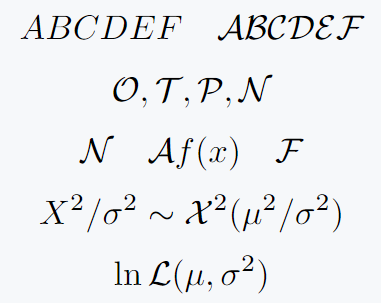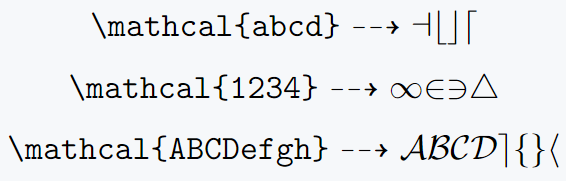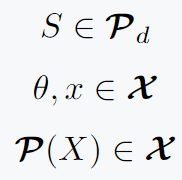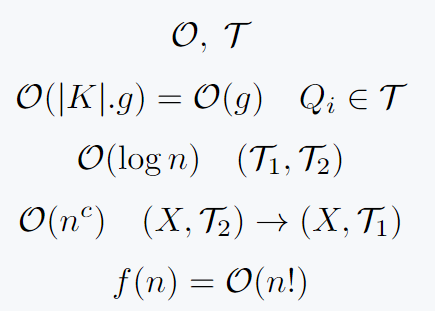# Use mathcal command for calligraphic font in LaTeX

You have come to the right place. In this content, the calligraphic font style is represented by \mathcal{} command.

For your convenience, various problems have been solved, which you face.

However, this command is only valid for math mode.

## Use mathcal command for Calligraphic font

Usage of this symbol is much more in terms of usage. From mathematically normal distribution to Big o notation and topology.

\documentclass{article}
\begin{document}
$ABCDEF \quad \mathcal{ABCDEF}$
$\mathcal{O},\mathcal{T},\mathcal{P},\mathcal{N}$
$\mathcal{N}\quad\mathcal{A}f(x)\quad\mathcal{F}$
$X^2/\sigma^2 \sim \mathcal{X}^2(\mu^2/\sigma^2)$
$\ln \mathcal{L}(\mu ,\sigma^2)$
\end{document}

Output :## Lowercase Calligraphic fonts

\mathcal{} commands have some limitations. Because even if the capital alphabet is converted to this font. Cannot convert digits and small alphabets to calligraphy fonts.

\documentclass{article}
\usepackage{amssymb}
\begin{document}
$\verb|\mathcal{abcd}|\dashrightarrow \mathcal{abcd}$
$\verb|\mathcal{1234}|\dashrightarrow\mathcal{1234}$
$\verb|\mathcal{ABCDefgh}|\dashrightarrow\mathcal{ABCDefgh}$
\end{document}

Output :## Calligraphic bold font style

You will see many commands that convert text or math expressions to bold font style like \textbf{}, \mathbm, bf etc.

However, in case of calligraphy font style, \boldsymbol should be used which will convert font style of symbol to bold.

Because if you use another command instead of \boldsymbol, output will show an arrow.

\documentclass{article}
\usepackage{amsmath}
\begin{document}
$S\in \boldsymbol{\mathcal{P}}_d$
$\theta,x\in\boldsymbol{\mathcal{X}}$
$\boldsymbol{\mathcal{P}}(X)\in\boldsymbol{\mathcal{X}}$
\end{document}

Output :## Big O notation and topology T denoted by mathcal command

Below are some examples of Big O notation and Topology T by this command.

\documentclass{article}
\begin{document}
$\mathcal{O},\;\mathcal{T}$
$\mathcal{O}(|K|.g)=\mathcal{O}(g)\quad Q_i\in \mathcal{T}$
$\mathcal{O}(\log n)\quad (\mathcal{T}_1 ,\mathcal{T}_2)$
$\mathcal{O}(n^c)\quad (X,\mathcal{T}_2)\rightarrow (X,\mathcal{T}_1)$
$f(n) = \mathcal{O}(n!)$
\end{document}

Output :#### Md Jidan Mondal

LaTeX expert with over 10 years of experience in document preparation and typesetting. Specializes in creating professional documents, reports, and presentations using LaTeX.